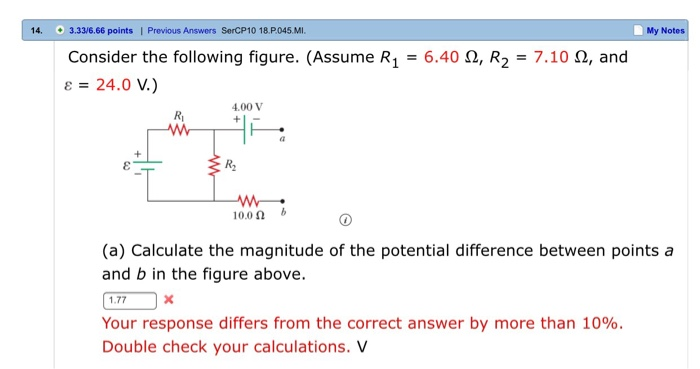# Solved: 14 3.33/6.66 Points | Previous Answers SerCP10 18.P045.MI. My Notes 6.40 , R2 Consider The Following Figure. (Assume R1 7.10 Q, And 8 24.0 V.) 4.00 V Ri 10.0 (a) Calculate The Magnitude Of The

By |14 3.33/6.66 points | Previous Answers SerCP10 18.P045.MI. My Notes 6.40 , R2 Consider the following figure. (Assume R1 7.10 Q, and 8 24.0 V.) 4.00 V Ri 10.0 (a) Calculate the magnitude of the potential difference between points a and b in the figure above. 1.77 Your response differs from the correct answer by more than 10%. Double check your calculations. V